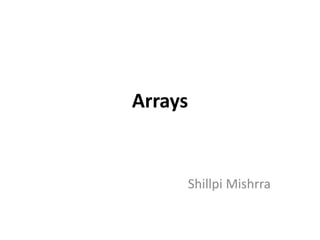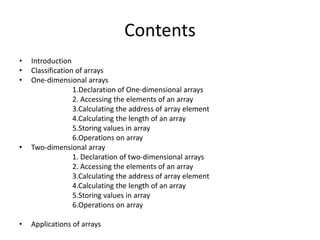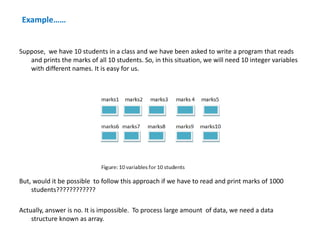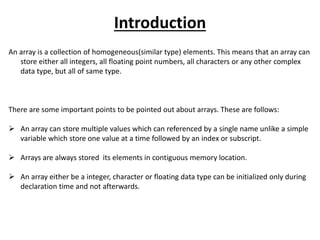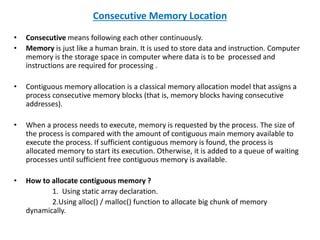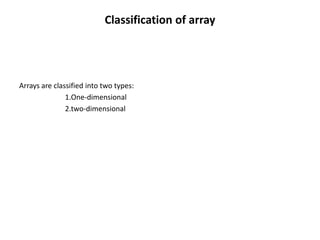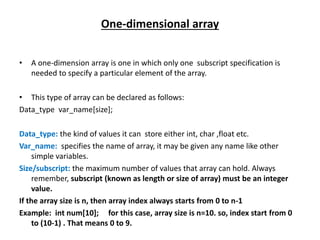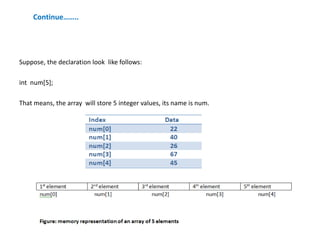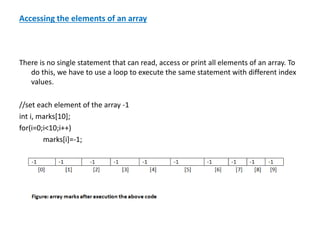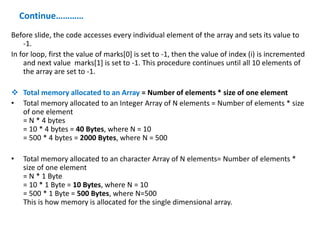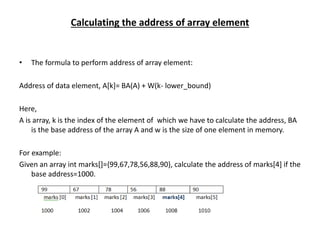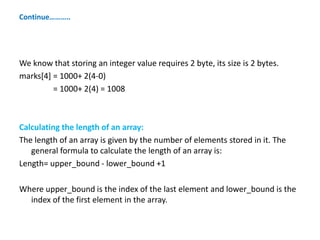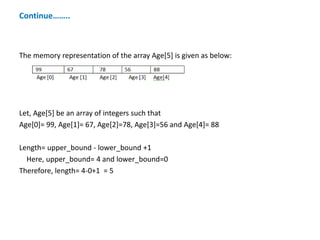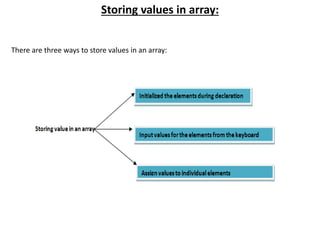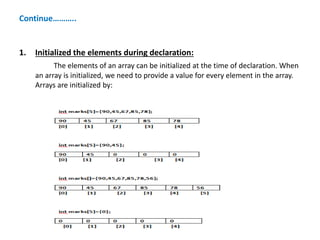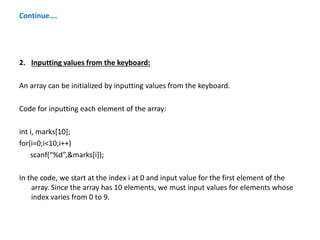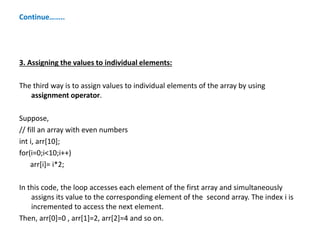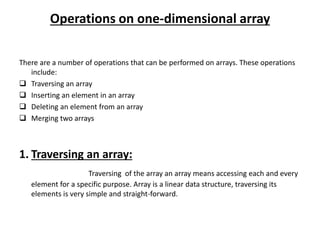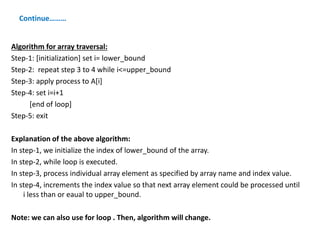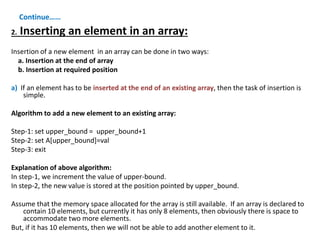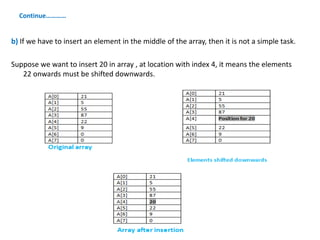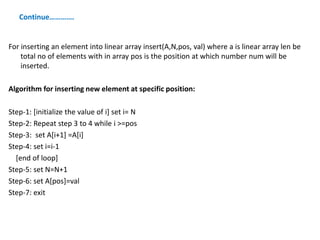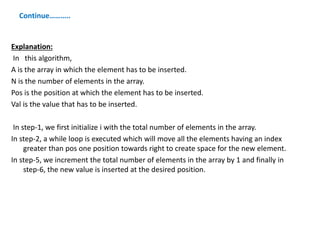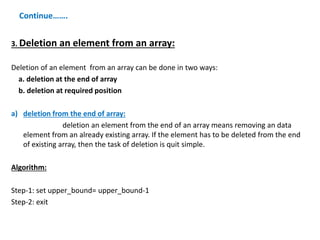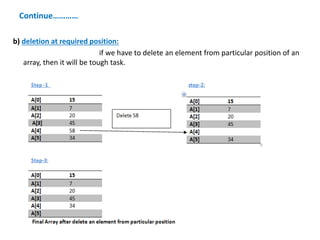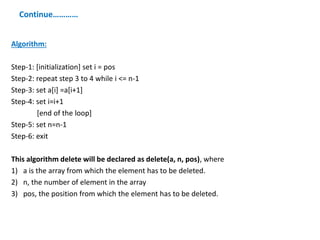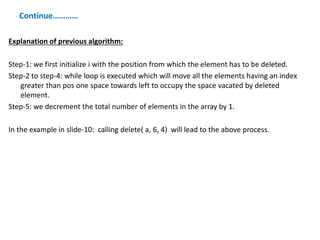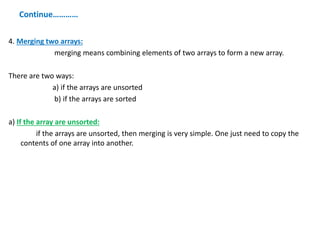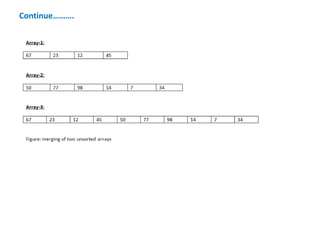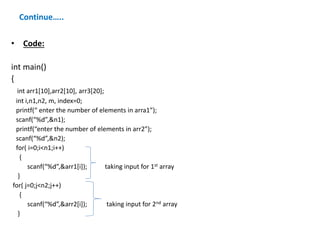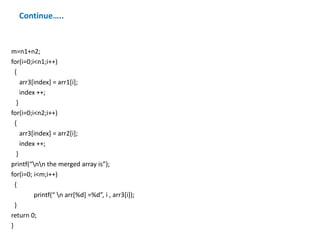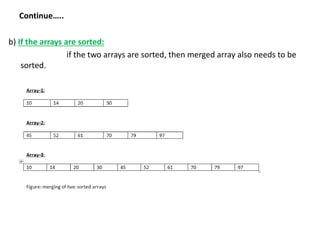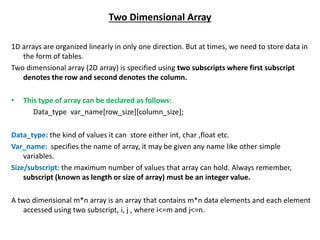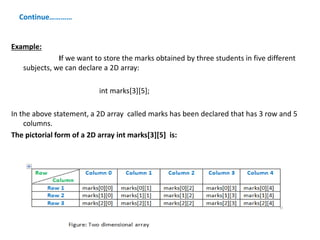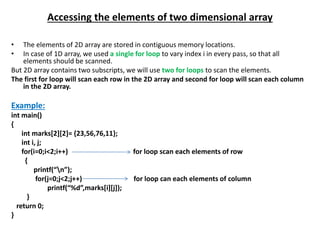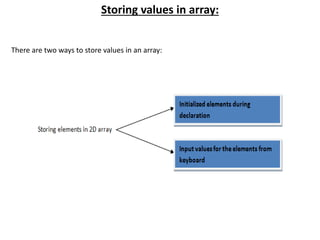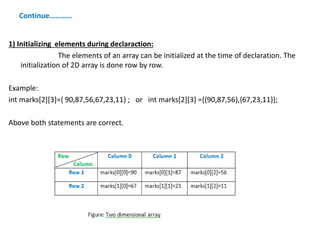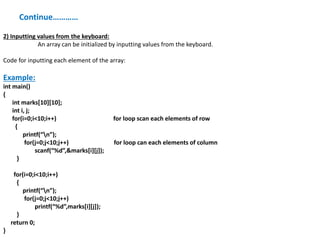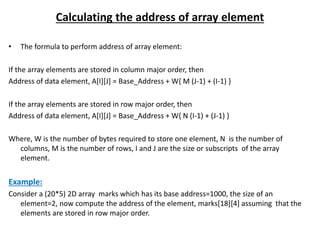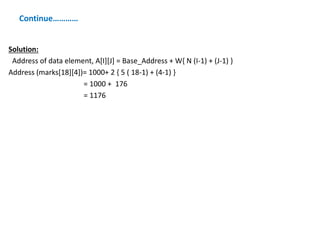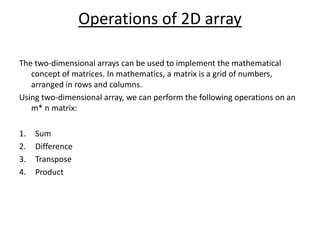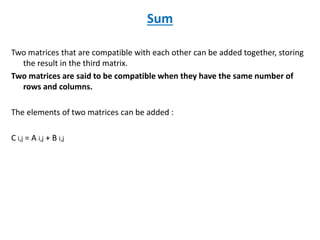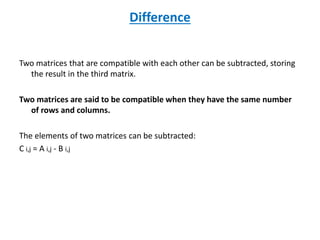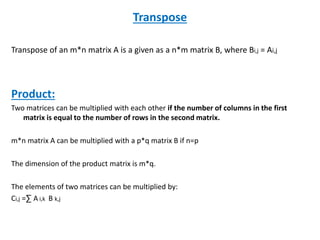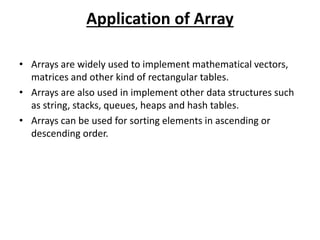1 de 45

### Arrays

1. Arrays Shillpi Mishrra
2. Contents • Introduction • Classification of arrays • One-dimensional arrays 1.Declaration of One-dimensional arrays 2. Accessing the elements of an array 3.Calculating the address of array element 4.Calculating the length of an array 5.Storing values in array 6.Operations on array • Two-dimensional array 1. Declaration of two-dimensional arrays 2. Accessing the elements of an array 3.Calculating the address of array element 4.Calculating the length of an array 5.Storing values in array 6.Operations on array • Applications of arrays
3. Example…… Suppose, we have 10 students in a class and we have been asked to write a program that reads and prints the marks of all 10 students. So, in this situation, we will need 10 integer variables with different names. It is easy for us. But, would it be possible to follow this approach if we have to read and print marks of 1000 students???????????? Actually, answer is no. It is impossible. To process large amount of data, we need a data structure known as array.
4. Introduction An array is a collection of homogeneous(similar type) elements. This means that an array can store either all integers, all floating point numbers, all characters or any other complex data type, but all of same type. There are some important points to be pointed out about arrays. These are follows:  An array can store multiple values which can referenced by a single name unlike a simple variable which store one value at a time followed by an index or subscript.  Arrays are always stored its elements in contiguous memory location.  An array either be a integer, character or floating data type can be initialized only during declaration time and not afterwards.
5. Consecutive Memory Location • Consecutive means following each other continuously. • Memory is just like a human brain. It is used to store data and instruction. Computer memory is the storage space in computer where data is to be processed and instructions are required for processing . • Contiguous memory allocation is a classical memory allocation model that assigns a process consecutive memory blocks (that is, memory blocks having consecutive addresses). • When a process needs to execute, memory is requested by the process. The size of the process is compared with the amount of contiguous main memory available to execute the process. If sufficient contiguous memory is found, the process is allocated memory to start its execution. Otherwise, it is added to a queue of waiting processes until sufficient free contiguous memory is available. • How to allocate contiguous memory ? 1. Using static array declaration. 2.Using alloc() / malloc() function to allocate big chunk of memory dynamically.
6. Classification of array Arrays are classified into two types: 1.One-dimensional 2.two-dimensional
7. One-dimensional array • A one-dimension array is one in which only one subscript specification is needed to specify a particular element of the array. • This type of array can be declared as follows: Data_type var_name[size]; Data_type: the kind of values it can store either int, char ,float etc. Var_name: specifies the name of array, it may be given any name like other simple variables. Size/subscript: the maximum number of values that array can hold. Always remember, subscript (known as length or size of array) must be an integer value. If the array size is n, then array index always starts from 0 to n-1 Example: int num; for this case, array size is n=10. so, index start from 0 to (10-1) . That means 0 to 9.
8. Continue…….. Suppose, the declaration look like follows: int num; That means, the array will store 5 integer values, its name is num.
9. Accessing the elements of an array There is no single statement that can read, access or print all elements of an array. To do this, we have to use a loop to execute the same statement with different index values. //set each element of the array -1 int i, marks; for(i=0;i<10;i++) marks[i]=-1;
10. Continue………… Before slide, the code accesses every individual element of the array and sets its value to -1. In for loop, first the value of marks is set to -1, then the value of index (i) is incremented and next value marks is set to -1. This procedure continues until all 10 elements of the array are set to -1.  Total memory allocated to an Array = Number of elements * size of one element • Total memory allocated to an Integer Array of N elements = Number of elements * size of one element = N * 4 bytes = 10 * 4 bytes = 40 Bytes, where N = 10 = 500 * 4 bytes = 2000 Bytes, where N = 500 • Total memory allocated to an character Array of N elements= Number of elements * size of one element = N * 1 Byte = 10 * 1 Byte = 10 Bytes, where N = 10 = 500 * 1 Byte = 500 Bytes, where N=500 This is how memory is allocated for the single dimensional array.
11. Calculating the address of array element • The formula to perform address of array element: Address of data element, A[k]= BA(A) + W(k- lower_bound) Here, A is array, k is the index of the element of which we have to calculate the address, BA is the base address of the array A and w is the size of one element in memory. For example: Given an array int marks[]={99,67,78,56,88,90}, calculate the address of marks if the base address=1000.
12. Continue……….. We know that storing an integer value requires 2 byte, its size is 2 bytes. marks = 1000+ 2(4-0) = 1000+ 2(4) = 1008 Calculating the length of an array: The length of an array is given by the number of elements stored in it. The general formula to calculate the length of an array is: Length= upper_bound - lower_bound +1 Where upper_bound is the index of the last element and lower_bound is the index of the first element in the array.
13. Continue…….. The memory representation of the array Age is given as below: Let, Age be an array of integers such that Age= 99, Age= 67, Age=78, Age=56 and Age= 88 Length= upper_bound - lower_bound +1 Here, upper_bound= 4 and lower_bound=0 Therefore, length= 4-0+1 = 5
14. Storing values in array: There are three ways to store values in an array:
15. Continue……….. 1. Initialized the elements during declaration: The elements of an array can be initialized at the time of declaration. When an array is initialized, we need to provide a value for every element in the array. Arrays are initialized by:
16. Continue…. 2. Inputting values from the keyboard: An array can be initialized by inputting values from the keyboard. Code for inputting each element of the array: int i, marks; for(i=0;i<10;i++) scanf(“%d”,&marks[i]); In the code, we start at the index i at 0 and input value for the first element of the array. Since the array has 10 elements, we must input values for elements whose index varies from 0 to 9.
17. Continue…….. 3. Assigning the values to individual elements: The third way is to assign values to individual elements of the array by using assignment operator. Suppose, // fill an array with even numbers int i, arr; for(i=0;i<10;i++) arr[i]= i*2; In this code, the loop accesses each element of the first array and simultaneously assigns its value to the corresponding element of the second array. The index i is incremented to access the next element. Then, arr=0 , arr=2, arr=4 and so on.
18. Operations on one-dimensional array There are a number of operations that can be performed on arrays. These operations include:  Traversing an array  Inserting an element in an array  Deleting an element from an array  Merging two arrays 1. Traversing an array: Traversing of the array an array means accessing each and every element for a specific purpose. Array is a linear data structure, traversing its elements is very simple and straight-forward.
19. Continue……… Algorithm for array traversal: Step-1: [initialization] set i= lower_bound Step-2: repeat step 3 to 4 while i<=upper_bound Step-3: apply process to A[i] Step-4: set i=i+1 [end of loop] Step-5: exit Explanation of the above algorithm: In step-1, we initialize the index of lower_bound of the array. In step-2, while loop is executed. In step-3, process individual array element as specified by array name and index value. In step-4, increments the index value so that next array element could be processed until i less than or eaual to upper_bound. Note: we can also use for loop . Then, algorithm will change.
20. Continue…… 2. Inserting an element in an array: Insertion of a new element in an array can be done in two ways: a. Insertion at the end of array b. Insertion at required position a) If an element has to be inserted at the end of an existing array, then the task of insertion is simple. Algorithm to add a new element to an existing array: Step-1: set upper_bound = upper_bound+1 Step-2: set A[upper_bound]=val Step-3: exit Explanation of above algorithm: In step-1, we increment the value of upper-bound. In step-2, the new value is stored at the position pointed by upper_bound. Assume that the memory space allocated for the array is still available. If an array is declared to contain 10 elements, but currently it has only 8 elements, then obviously there is space to accommodate two more elements. But, if it has 10 elements, then we will not be able to add another element to it.
21. Continue………… b) If we have to insert an element in the middle of the array, then it is not a simple task. Suppose we want to insert 20 in array , at location with index 4, it means the elements 22 onwards must be shifted downwards.
22. Continue…………. For inserting an element into linear array insert(A,N,pos, val) where a is linear array len be total no of elements with in array pos is the position at which number num will be inserted. Algorithm for inserting new element at specific position: Step-1: [initialize the value of i] set i= N Step-2: Repeat step 3 to 4 while i >=pos Step-3: set A[i+1] =A[i] Step-4: set i=i-1 [end of loop] Step-5: set N=N+1 Step-6: set A[pos]=val Step-7: exit
23. Continue……….. Explanation: In this algorithm, A is the array in which the element has to be inserted. N is the number of elements in the array. Pos is the position at which the element has to be inserted. Val is the value that has to be inserted. In step-1, we first initialize i with the total number of elements in the array. In step-2, a while loop is executed which will move all the elements having an index greater than pos one position towards right to create space for the new element. In step-5, we increment the total number of elements in the array by 1 and finally in step-6, the new value is inserted at the desired position.
24. Continue……. 3. Deletion an element from an array: Deletion of an element from an array can be done in two ways: a. deletion at the end of array b. deletion at required position a) deletion from the end of array: deletion an element from the end of an array means removing an data element from an already existing array. If the element has to be deleted from the end of existing array, then the task of deletion is quit simple. Algorithm: Step-1: set upper_bound= upper_bound-1 Step-2: exit
25. Continue………… b) deletion at required position: if we have to delete an element from particular position of an array, then it will be tough task.
26. Continue………… Algorithm: Step-1: [initialization] set i = pos Step-2: repeat step 3 to 4 while i <= n-1 Step-3: set a[i] =a[i+1] Step-4: set i=i+1 [end of the loop] Step-5: set n=n-1 Step-6: exit This algorithm delete will be declared as delete(a, n, pos), where 1) a is the array from which the element has to be deleted. 2) n, the number of element in the array 3) pos, the position from which the element has to be deleted.
27. Continue………… Explanation of previous algorithm: Step-1: we first initialize i with the position from which the element has to be deleted. Step-2 to step-4: while loop is executed which will move all the elements having an index greater than pos one space towards left to occupy the space vacated by deleted element. Step-5: we decrement the total number of elements in the array by 1. In the example in slide-10: calling delete( a, 6, 4) will lead to the above process.
28. Continue………… 4. Merging two arrays: merging means combining elements of two arrays to form a new array. There are two ways: a) if the arrays are unsorted b) if the arrays are sorted a) If the array are unsorted: if the arrays are unsorted, then merging is very simple. One just need to copy the contents of one array into another.
29. Continue……….
30. Continue….. • Code: int main() { int arr1,arr2, arr3; int i,n1,n2, m, index=0; printf(“ enter the number of elements in arra1”); scanf(“%d”,&n1); printf(“enter the number of elements in arr2”); scanf(“%d”,&n2); for( i=0;i<n1;i++) { scanf(“%d”,&arr1[i]); taking input for 1st array } for( j=0;j<n2;j++) { scanf(“%d”,&arr2[i]); taking input for 2nd array }
31. Continue….. m=n1+n2; for(i=0;i<n1;i++) { arr3[index] = arr1[i]; index ++; } for(i=0;i<n2;i++) { arr3[index] = arr2[i]; index ++; } printf(“nn the merged array is”); for(i=0; i<m;i++) { printf(“ n arr[%d] =%d”, i , arr3[i]); } return 0; }
32. Continue….. b) If the arrays are sorted: if the two arrays are sorted, then merged array also needs to be sorted.
33. Two Dimensional Array 1D arrays are organized linearly in only one direction. But at times, we need to store data in the form of tables. Two dimensional array (2D array) is specified using two subscripts where first subscript denotes the row and second denotes the column. • This type of array can be declared as follows: Data_type var_name[row_size][column_size]; Data_type: the kind of values it can store either int, char ,float etc. Var_name: specifies the name of array, it may be given any name like other simple variables. Size/subscript: the maximum number of values that array can hold. Always remember, subscript (known as length or size of array) must be an integer value. A two dimensional m*n array is an array that contains m*n data elements and each element accessed using two subscript, i, j , where i<=m and j<=n.
34. Continue………… Example: If we want to store the marks obtained by three students in five different subjects, we can declare a 2D array: int marks; In the above statement, a 2D array called marks has been declared that has 3 row and 5 columns. The pictorial form of a 2D array int marks is:
35. Accessing the elements of two dimensional array • The elements of 2D array are stored in contiguous memory locations. • In case of 1D array, we used a single for loop to vary index i in every pass, so that all elements should be scanned. But 2D array contains two subscripts, we will use two for loops to scan the elements. The first for loop will scan each row in the 2D array and second for loop will scan each column in the 2D array. Example: int main() { int marks= {23,56,76,11}; int i, j; for(i=0;i<2;i++) for loop scan each elements of row { printf(“n”); for(j=0;j<2;j++) for loop can each elements of column printf(“%d”,marks[i][j]); } return 0; }
36. Storing values in array: There are two ways to store values in an array:
37. Continue………… 1) Initializing elements during declaraction: The elements of an array can be initialized at the time of declaration. The initialization of 2D array is done row by row. Example: int marks={ 90,87,56,67,23,11} ; or int marks ={{90,87,56},{67,23,11}}; Above both statements are correct.
38. Continue………… 2) Inputting values from the keyboard: An array can be initialized by inputting values from the keyboard. Code for inputting each element of the array: Example: int main() { int marks; int i, j; for(i=0;i<10;i++) for loop scan each elements of row { printf(“n”); for(j=0;j<10;j++) for loop can each elements of column scanf(“%d”,&marks[i][j]); } for(i=0;i<10;i++) { printf(“n”); for(j=0;j<10;j++) printf(“%d”,marks[i][j]); } return 0; }
39. Calculating the address of array element • The formula to perform address of array element: If the array elements are stored in column major order, then Address of data element, A[I][J] = Base_Address + W{ M (J-1) + (I-1) } If the array elements are stored in row major order, then Address of data element, A[I][J] = Base_Address + W{ N (I-1) + (J-1) } Where, W is the number of bytes required to store one element, N is the number of columns, M is the number of rows, I and J are the size or subscripts of the array element. Example: Consider a (20*5) 2D array marks which has its base address=1000, the size of an element=2, now compute the address of the element, marks assuming that the elements are stored in row major order.
40. Continue………… Solution: Address of data element, A[I][J] = Base_Address + W{ N (I-1) + (J-1) } Address (marks)= 1000+ 2 { 5 ( 18-1) + (4-1) } = 1000 + 176 = 1176
41. Operations of 2D array The two-dimensional arrays can be used to implement the mathematical concept of matrices. In mathematics, a matrix is a grid of numbers, arranged in rows and columns. Using two-dimensional array, we can perform the following operations on an m* n matrix: 1. Sum 2. Difference 3. Transpose 4. Product
42. Sum Two matrices that are compatible with each other can be added together, storing the result in the third matrix. Two matrices are said to be compatible when they have the same number of rows and columns. The elements of two matrices can be added : C i,j = A i,j + B i,j
43. Difference Two matrices that are compatible with each other can be subtracted, storing the result in the third matrix. Two matrices are said to be compatible when they have the same number of rows and columns. The elements of two matrices can be subtracted: C i,j = A i,j - B i,j
44. Transpose Transpose of an m*n matrix A is a given as a n*m matrix B, where Bi,j = Ai,j Product: Two matrices can be multiplied with each other if the number of columns in the first matrix is equal to the number of rows in the second matrix. m*n matrix A can be multiplied with a p*q matrix B if n=p The dimension of the product matrix is m*q. The elements of two matrices can be multiplied by: Ci,j =∑ A i,k B k,j
45. Application of Array • Arrays are widely used to implement mathematical vectors, matrices and other kind of rectangular tables. • Arrays are also used in implement other data structures such as string, stacks, queues, heaps and hash tables. • Arrays can be used for sorting elements in ascending or descending order.